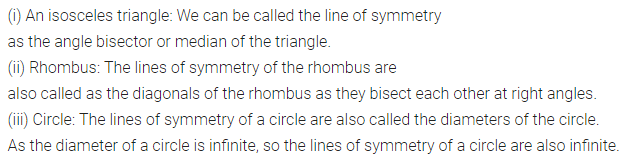ML Aggarwal Class 7 Solutions Chapter 14 Symmetry Ex 14.1 for ICSE Understanding Mathematics acts as the best resource during your learning and helps you score well in your exams.

## ML Aggarwal Class 7 Solutions for ICSE Maths Chapter 14 Symmetry Ex 14.1

Question 1.
Draw all lines of symmetry, if any, in each of the following figures:Solution: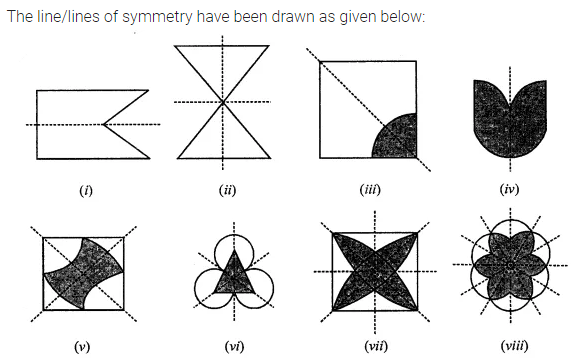Question 2.
Copy the figures with a punched hole(s) and draw all the axes of symmetry in each of the following: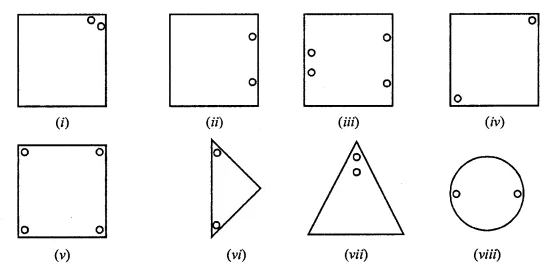Solution: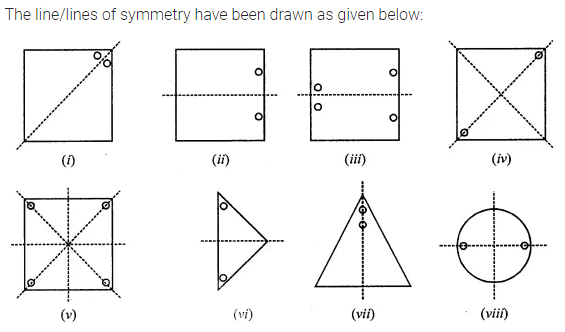Question 3.
In the following figure, mark the missing hole(s) in order to make them symmetrical about the dotted line: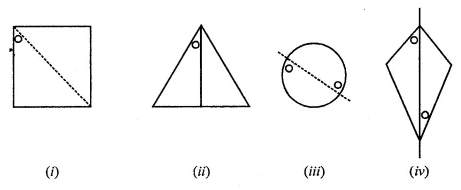Solution: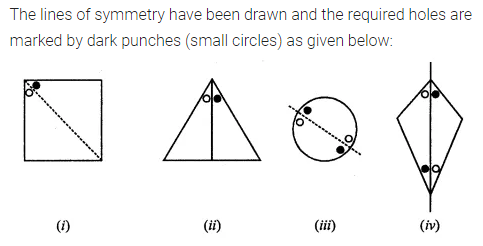Question 4.
In the following figures, the mirror line (line of symmetry) is given as dotted line. Complete each figure by performing reflection in the mirror (dotted) line and name the figure you complete:Solution:Question 5.
Take any one diagonal as a line of symmetry and shade a few more squares to make the figure symmetric about a diagonal. Is there more than one way to do that? Will the figure be symmetric about both the diagonals?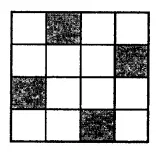Solution:Question 6.
Draw the reflection of the following figures/letter in the given mirror line shown dotted:Solution:Question 7.
What other names can you give to the line of symmetry of
(i) an isosceles triangle
(ii) rhombus
(iii) circle?
Solution: